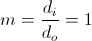# MCAT Physical : Magnification

## Example Questions

### Example Question #1 : Mirrors And Lenses

A person approaches a plane mirror at 5m/s. How fast do they approach the mirror image?

5m/s

2.5m/s

7.5m/s

10m/s

10m/s

Explanation:

The image distance for a plane mirror is always equal to the object distance because the magnification is 1.If the object and image are the same distance from the mirror and magnification is 1, then as the object approaches the mirror at a certain speed, the image is approaching the plane mirror at the same speed, therefore you approach the image more quickly than you approach the mirror, since you travel 5m/s toward the mirror and the image travels 5m/s toward the mirror.

### All MCAT Physical Resources